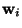Next: Algorithms for ICA Up: Objective (Contrast) Functions for Previous: General contrast functions

## A unifying view on contrast functions

It is possible to give a unifying view that encompasses most of the important contrast functions for ICA.

First of all, we saw above that the principles of mutual information and maximum likelihood are essentially equivalent . Second, as already discussed above, the infomax principle is equivalent to maximum likelihood estimation [23,123]. The nonlinear PCA criteria can be shown to be equivalent to maximum likelihood estimation as well . On the other hand, it was discussed above how some of the cumulant-based contrasts can be considered as approximations of mutual information. Thus it can be seen that most of the multi-unit contrast functions are, if not strictly equivalent, at least very closely related.

However, an important reservation is necessary here: for these equivalencies to be at all valid, the densities fi used in the likelihood must be a sufficiently good approximations of the true densities of the independent components. At the minimum, we must have one bit of information on each independent component: whether it is sub- or super-Gaussian [28,26,73]. This information must be either available a priori or estimated from the data, see [28,26,96,124]. This situation is quite different with most contrast functions based on cumulants, and the general contrast functions in Section 4.4.3, which estimate directly independent components of almost any non-Gaussian distribution.

As for the one-unit contrast functions, we have a very similar situation. Negentropy can be approximated by cumulants, or the general contrast functions in Section 4.4.3, which shows that the considered contrast functions are very closely related.

In fact, looking at the formulas for likelihood and mutual information in (13) and (16), one sees that they can be considered as sums of one-unit contrast functions plus a penalizing term that prevents the vectorsfrom converging to the same directions. This could be called a 'soft' form of decorrelation. Thus we see that almost all the contrast functions could be described by the single intuitive principle: Find the most nongaussian projections, and use some (soft) decorrelation to make sure that different independent components are found.

So, the choice of contrast function is essentially reduced the simple choice between estimating all the independent components in parallel, or just estimating a few of them (possibly one-by-one). This corresponds approximately to the choosing between symmetric and hierachical decorrelation, which is a choice familiar in PCA learning [114,111].

One must also make the less important choice between cumulant-based and robust contrast functions (i.e. those based on nonquadratic functions as in (29)), but it seems that the robust contrast functions are to be preferred in most applications.Next: Algorithms for ICA Up: Objective (Contrast) Functions for Previous: General contrast functions
Aapo Hyvarinen
1999-04-23